# Long-Term EU and US GDP Growth Rate by Region, Fourth Quarter 2018

Aug 6, 2019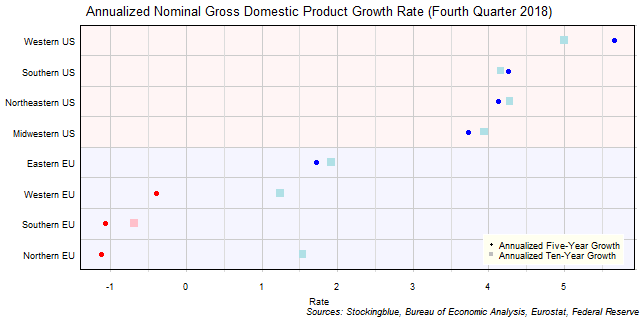The chart above shows the annualized nominal gross domestic product (GDP) growth rate when priced in US dollars in each EU and US region over the past five years as of the fourth quarter of 2018 and the growth over the past ten years.  All negative growth rates in the EU are attributed to currency rate fluctuations.

# Long-Term EU GDP Growth Rate by Region, Fourth Quarter 2018

Aug 5, 2019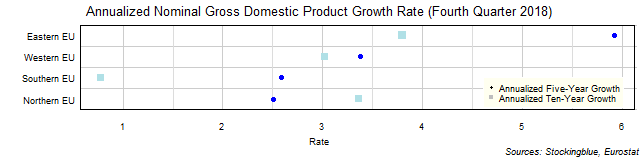The chart above shows the annualized nominal gross domestic product (GDP) growth rate in each EU region over the past five years as of the fourth quarter of 2018 and the growth rate over the past ten years.  The Northern EU is the only region whose growth rate was smaller over the past five years than it was over the past ten years.

# Long-Term US GDP Growth Rate by Region, Fourth Quarter 2018

Aug 2, 2019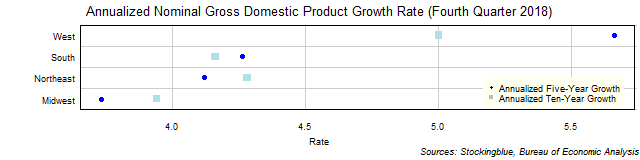The chart above shows the annualized nominal gross domestic product (GDP) growth rate in each US region over the past five years as of the fourth quarter of 2018 and the growth rate over the past ten years.  The West's growth rates outshine the other regions' rates.

# Long-Term EU and US GDP Growth Rate by State, Fourth Quarter 2018

Aug 1, 2019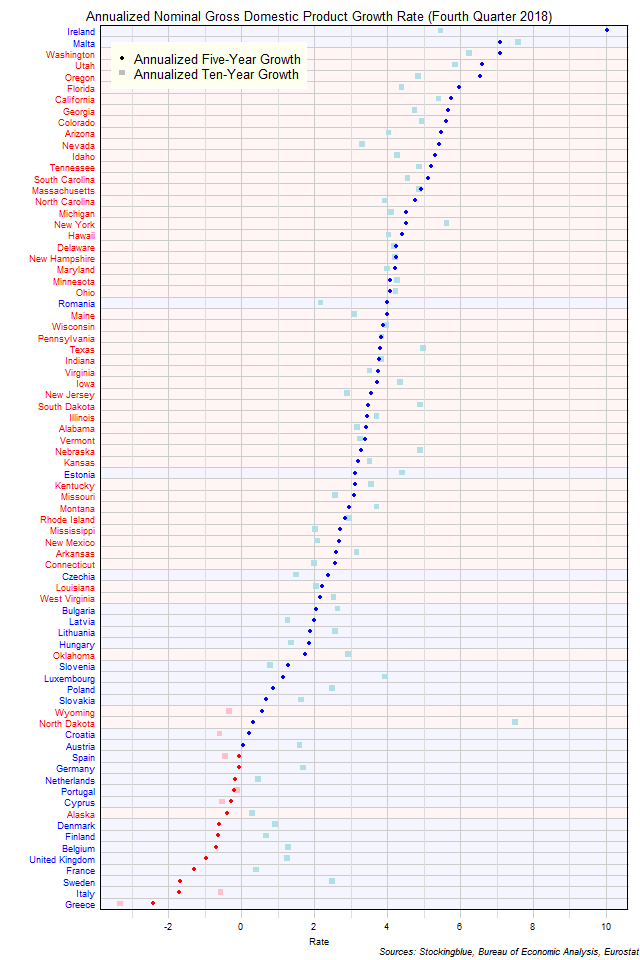The chart above shows the annualized nominal gross domestic product (GDP) growth rate when the GDP is priced in US dollars in each EU and US state over the past five years as of the fourth quarter of 2018 and the growth rate over the past ten years.  Only EU states saw a contraction both over the past five and ten years.

# Long-Term EU GDP Growth Rate by State, Fourth Quarter 2018

Jul 31, 2019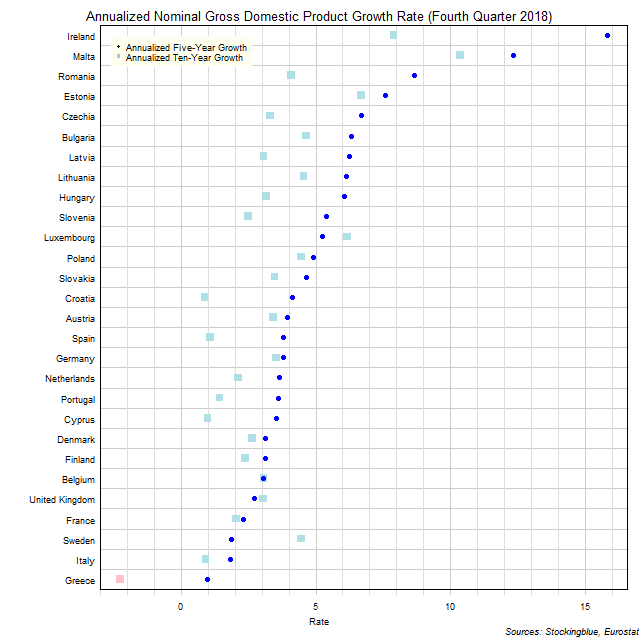The chart above shows the annualized nominal gross domestic product (GDP) growth rate in each EU state over the past five years as of the fourth quarter of 2018 and the growth rate over the past ten years.  Greece is the only state to experience negative growth over either period.

# Long-Term US GDP Growth Rate by State, Fourth Quarter 2018

Jul 30, 2019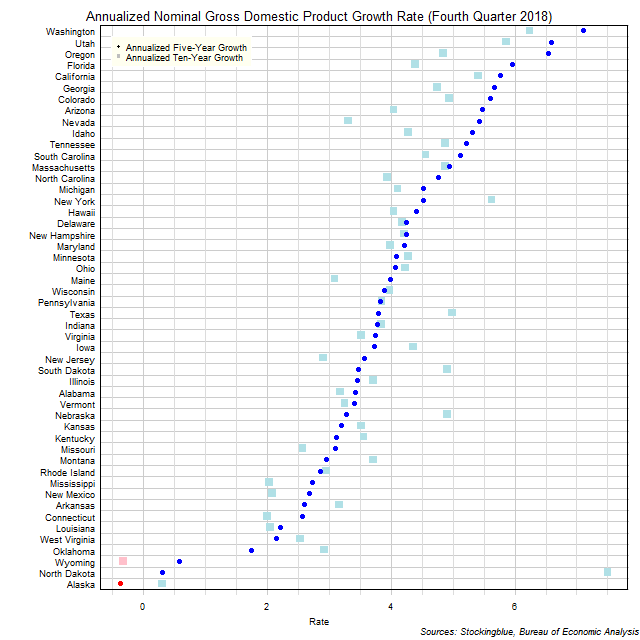The chart above shows the annualized nominal gross domestic product (GDP) growth rate in each US state over the past five years as of the fourth quarter of 2018 and the growth rate over the past ten years.  Only two states experienced negative growth in either time period.

# Long-Term Per Capita GDP by EU and US Region, Fourth Quarter 2018

Jul 29, 2019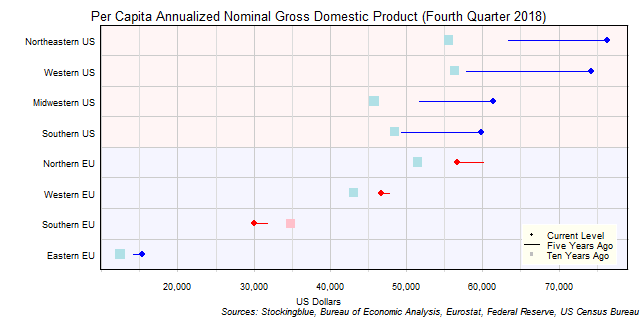The chart above shows the per capita annualized nominal gross domestic product (GDP) in each EU and US region as of the fourth quarter of 2018 in US dollars, the change from five years ago, and the per capita GDP ten years prior.  US regions have had a very good past five years.

# Long-Term Per Capita GDP by EU Region, Fourth Quarter 2018

Jul 26, 2019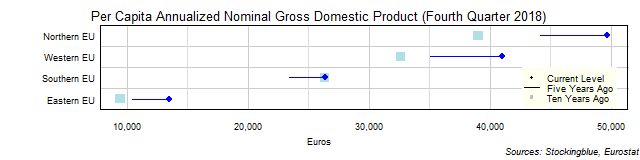The chart above shows the per capita annualized nominal gross domestic product (GDP) in each EU region as of the fourth quarter of 2018 in euros, the change from five years ago, and the per capita GDP ten years prior.  Every single region's economy grew both over the past quarter and the past year.

# Long-Term Per Capita GDP by US Region, Fourth Quarter 2018

Jul 25, 2019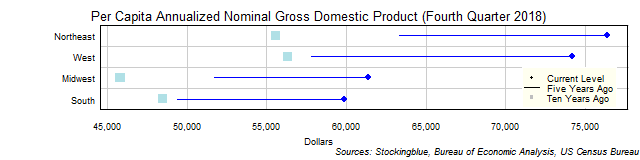The chart above shows the per capita annualized nominal gross domestic product (GDP) in each US region as of the fourth quarter of 2018 in dollars, the change from five years ago, and the per capita GDP ten years prior.  Every single region's economy grew both over the past five and past ten years.

# Long-Term Per Capita GDP by EU and US State, Fourth Quarter 2018

Jul 24, 2019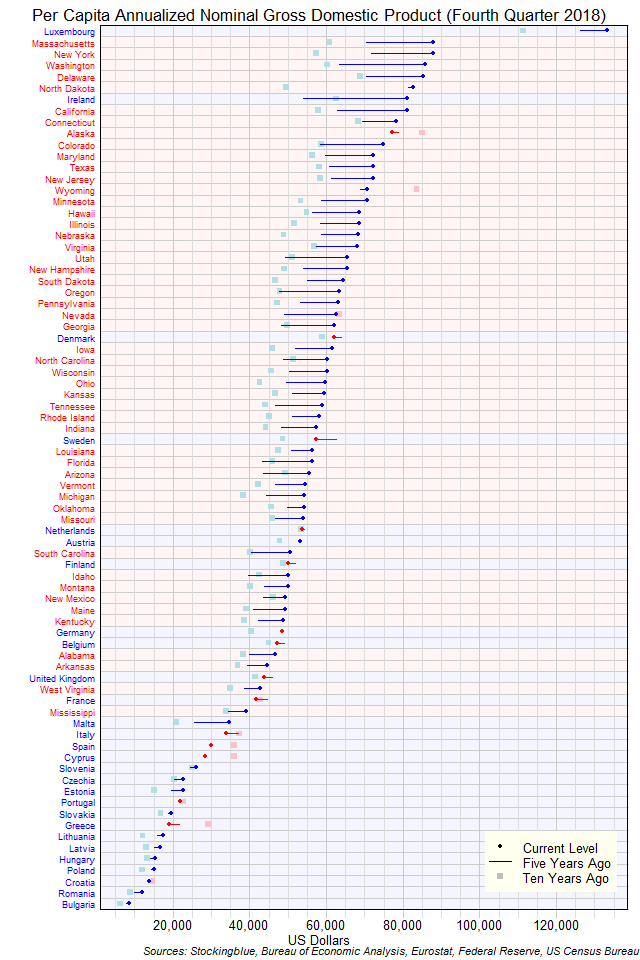The chart above shows the per capita annualized nominal gross domestic product (GDP) in each EU and US state as of the fourth quarter of 2018 in dollars, the change from five years ago, and the GDP ten years prior.  Luxembourg is in a class all by itself.

OlderNewer